# PSEB 4th Class Maths Solutions Chapter 8 Perimeter and Area Ex 8.1

Punjab State Board PSEB 4th Class Maths Book Solutions Chapter 8 Perimeter and Area Ex 8.1 Textbook Exercise Questions and Answers.

## PSEB Solutions for Class 4 Maths Chapter 8 Perimeter and Area Ex 8.1

Question 1.
Find the perimeter of given figures:
(a)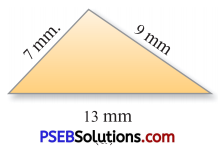Solution:
Perimeter of Figure = Sum of all sides of the figure
= 7 mm + 9 mm + 13 mm
= 29 mm

(b)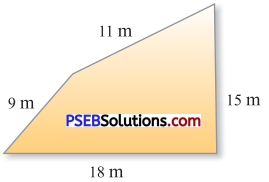Solution:
Perimeter of Figure = Sum of all sides of the figure
= 9 m + 11 m + 15 m + 18 m = 53 m.

(c)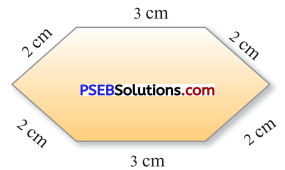Solution:
Perimeter of Figure = Sum of all sides of the figure = 2 cm + 2 cm + 3 cm + 3 cm + 2 cm + 2 cm
= 14 cmQuestion 2.
Find the perimeter of given figures :
(a)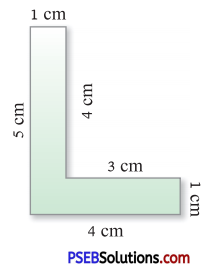Solution:
Perimeter of Figure
= Sum of all sides of the figure = 1 cm + 1 cm + 3 cm + 4 cm + 5 cm + 4 cm = 18 cm

(b)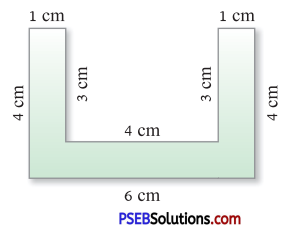Solution:
Perimeter of Figure
= Sum of all sides of the figure = 1 cm + 4 cm + 6 cm + 4 cm + 1 cm + 3 cm + 4 cm + 3 cm = 26 cm

(c)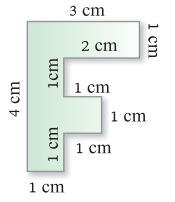Solution:
Perimeter of Figure
= Sum of all sides of the figure = 1 cm +’ 1 cm + 1 cm + 1 cm + 1 cm + 1 cm + 2 cm + 1 cm+ 3 cm + 4 cm = 16 cm

Question 3.
Find the perimeter of given figures :
(a)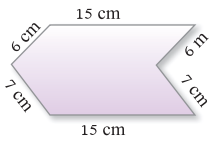Solution:
Perimeter of Figure
= Sum of all sides of the figure
= 6 cm + 7 cm + 15 cm + 6 cm 4 + 7 cm + 15 cm
= 56 cm

(b)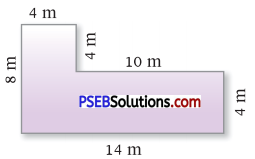Solution:
Perimeter of Figure
= Sum of all sides of the figure
= 4m+8m + 14m + 4m + 10m + 4m = 44 m

(c)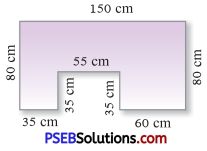Solution:
Perimeter of Figure
= Sum of all sides of the figure
= 35 cm + 35 cm + 55 cm + 35 cm + 60 cm + 80 cm + 150 cm + 80 cm
= 530 cm

(d)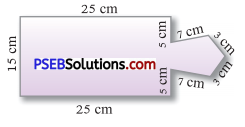Solution:
Perimeter of Figure
= Sum of all sides of the figure
= 15 cm + 25 cm + 5 cm + 7 cm + 3 cm + 3 cm + 7 cm + 5 cm + 25 cm
= 95 cmQuestion 4.
In given figures, the perimeter of which figure is less and by how much ?
(a)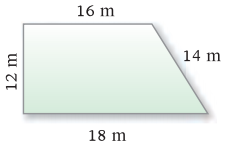Solution:
Perimeter of Figure (a)
= 12 m + 16 m + 14 m + 18 m = 60 m

(b)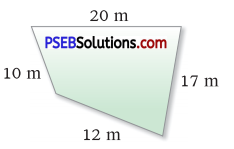Solution:
Perimeter of Figure (b)
= 10 m + 12 m + 17 m + 20 m = 59 m
Perimeter of Figure (b) is less than figurea (a) by =
(60 m – 59 m) = 1 m
Perimeter of Figure (b) is less by 1 m

Question 5.
Find the length of side with (?) of the given figures :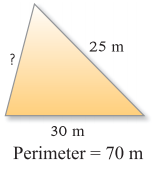Solution:
(a) Sides of the figure
= 30 m, 25 m and x m
Perimeter of the figure = 70 m
Length of the Side of the figure (x) = Perimeter – Sum of the two sides
x = 70 m – 55 m = 15 m.

(b) Sides of the figure = 34 cm, 43 cm
50 cm and x cm
Perimeter of the figure = 150 cm
Length of the Side of the figure (x) = Perimeter – Sum of the other three sides
x = 150 cm – (34 cm + 43 cm + 50 cm)
= 150 cm – 127 cm
= 23 cm

(c) Sides of the figure = 32 m, 68 m, 25 m,
37 m and x m.
Perimeter of the figure = 207 m
Length of the Side of the figure (x) = Perimeter – Sum of the other four sides
= 207 m – (32 m + 68 m + 25 m + 37 m)
= 207 m – 162 m = 45 mQuestion 6.
(a) Four sides of a Geld are 40 m, 35 m, 25 m and 28 m. Find its perimeter.
Solution:
Four sides of the field = 40 m, 35 m 25 m and 28 m
Perimeter of the field
= Sum of all sides
= 40m+35m+25m+28m
= 128m

(b) Length and breadth of a tennis court are 25 m and 9 m respectively. A net is required on four sides of the tennis court so that players do not face any difficulty. What is the length of the net required to cover the 4 sides of tennis court ?
Solution:
Length of the tennis court = 25 m
Breadth of the tennis court = 9 m
Perimeter of the tennis court = Length + Length + Breadth + Breadth
= 25 m + 25 m + 9 m + 9 m
= 68 m
The length of the net required to cover the 4 sides of tennis court = 68 m